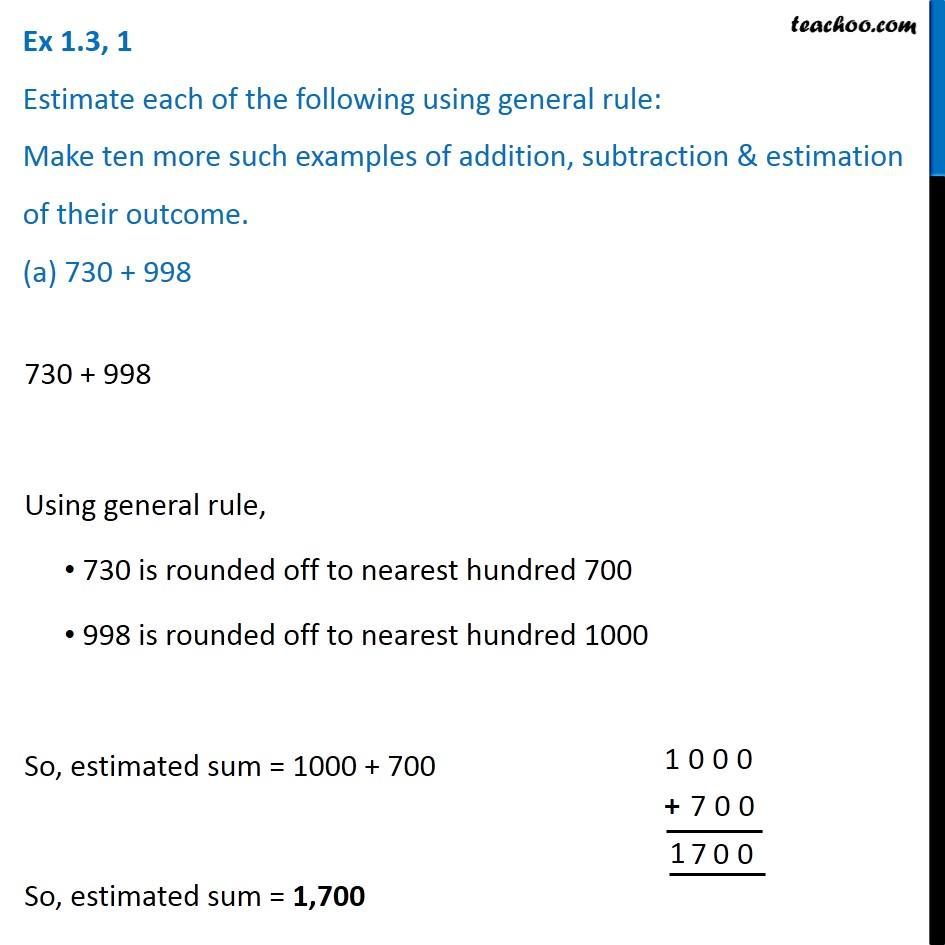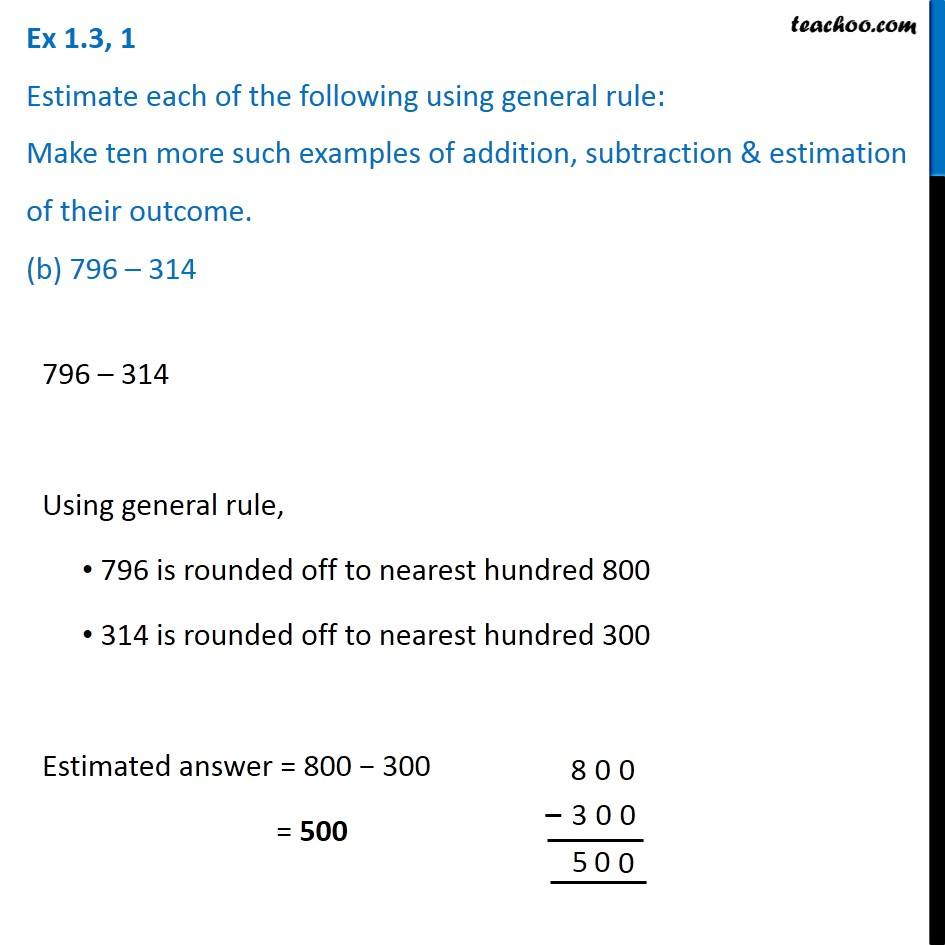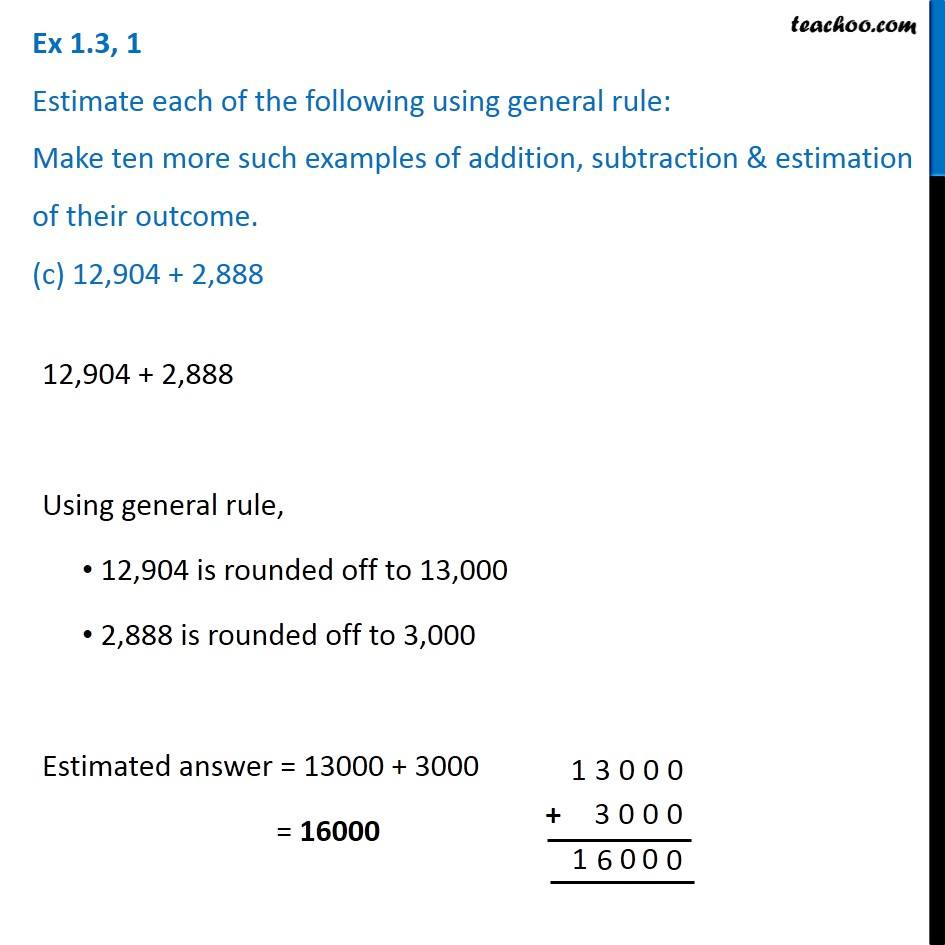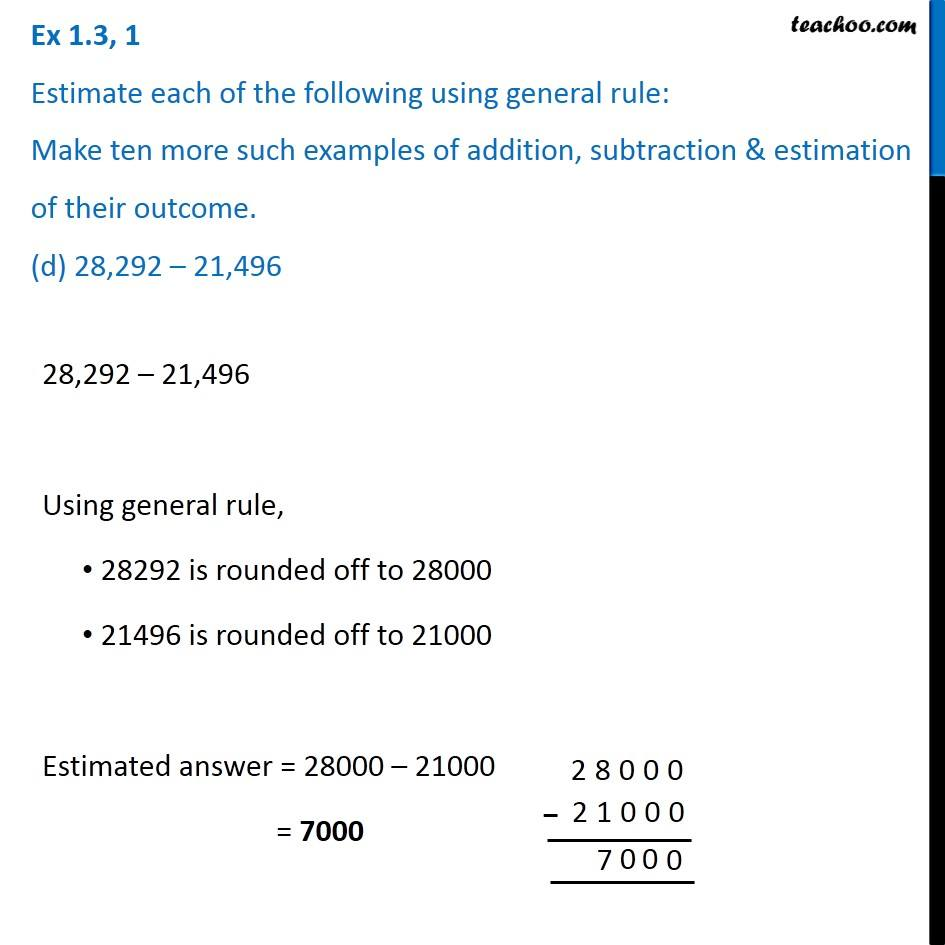Subscribe to our Youtube Channel - https://you.tube/teachoo

1. Chapter 1 Class 6 Knowing our Numbers
2. Serial order wise
3. Ex 1.3

Transcript

Ex 1.3, 1 Estimate each of the following using general rule: Make ten more such examples of addition, subtraction & estimation of their outcome. (a) 730 + 998 730 + 998 Using general rule, 730 is rounded off to nearest hundred 700 998 is rounded off to nearest hundred 1000 So, estimated sum = 1000 + 700 So, estimated sum = 1,700 Ex 1.3, 1 Estimate each of the following using general rule: Make ten more such examples of addition, subtraction & estimation of their outcome. (b) 796 – 314 796 – 314 Using general rule, 796 is rounded off to nearest hundred 800 314 is rounded off to nearest hundred 300 Estimated answer = 800 − 300 = 500 Ex 1.3, 1 Estimate each of the following using general rule: Make ten more such examples of addition, subtraction & estimation of their outcome. (c) 12,904 + 2,88812,904 + 2,888 Using general rule, 12,904 is rounded off to 13,000 2,888 is rounded off to 3,000 Estimated answer = 13000 + 3000 = 16000 Ex 1.3, 1 Estimate each of the following using general rule: Make ten more such examples of addition, subtraction & estimation of their outcome. (d) 28,292 – 21,49628,292 – 21,496 Using general rule, 28292 is rounded off to 28000 21496 is rounded off to 21000 Estimated answer = 28000 – 21000 = 7000

Ex 1.3